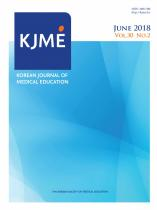상세검색
최근 검색어 전체 삭제
다국어입력
즐겨찾기0KCI우수등재 학술저널

# 의과대학 한 과목 시험에서 문항반응이론에 따른 검사 동등화 예Test Equating of a Medical School Lecture Examination Based on Item Response Theory: A Case Study

• 등재여부 : KCI우수등재
• 2005.04
• 15 - 28 (14 pages)
• 17

Purpose: To determine possible differences in the ability of students from two consecutive medical school class years, test equating based on item response theory was performed on the results of a lecture examination. Methods: The dataset for this study of the results of a medical school lecture exam was composed of the number of items and examinees: 75 students tested on 60 items in 2002, and 82 students tested on 50 items in 2003. We used common item non-equivalent group design and tested the assumption of unidimensionality and data-fitness. Item parameter estimates were based on the Rasch model, and the scale transformation was performed using mean/sigma moment methods. Equating was applied to both true and observed scores, and the results were analyzed. Results: The slope and intercept for the scale transformation of the 2003 exam to the 2002 exam were 1.2796 and 0.9630, respectively. The mean and standard deviation of the ability parameter of the students taking the 2003 exam were 1.69 and 0.66, respectively. After scale transformation, the mean increased to 3.13 and the standard deviation increased to 0.85. For the 2002 exam, the mean was 0.70 and the standard deviation was 0.66. The correlation coefficient between the true and observed scores was 0.9994 (P = 0.00). Conclusion: The difference in the ability parameter of students between the two years increased after scale transformation. Both the true and observed scores could be used interchangeably after equating. Thus, test equating based on item response theory may be applicable to medical school lecture examinations.

서 론

대상 및 방법

결 과

고 찰

참 고 문 헌